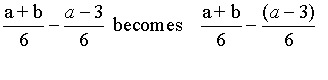### algebraic fractions

Create A Least Common DenominatorCreate Equivalent FractionsReducing FractionsPlacement of the Negative Sign in A FractionComputational TricksUse Parenthises To Simiplify The ExpressionAdd or Subtract FractionsDivide FractionsSome Problems and AnswersComputational Tricks
Rewrite problem with parentheses before you begin.When creating equivalent fractions, use pairs of parentheses
to make room for needed factors: ()() - ()()Create Equivalent Fractions
When creating equivalent fractions, think:
I have ...
I need ...
What's missing?

Reducing Fractions
Recall arithmeticDo the same with algebraic expressionsNegative Fractions
```
- 5        5	  5
-----  = ----- = - ----
3       - 3       3

The first and the last are prefered.	```

Use Parenthises To Simiplify The Expression
Rewrite problem with parentheses before you begin.1st: Put parenthises around the numerators.
2nd: Factor each denominator writing the factors below the denominator.
3rd: Use Common Factors First Method or One Fraction At-A-Time Method for finding a common denominator.
Alt. 3rd: Use these factors to create the common denominator by listing the factor of each denominator that are not duplicated in another fraction.
"I have ...", "I need ... "
4th: Recopy numerators leaving "holes" with parenthesis
5th: Rewrite the numerator with missing factors to create equivalent fractions.
"I have ...", "I need ... "
6th: Simplify numerator (top of fraction).
7th: Factor the numerator if possible.
8th: Reduce the fraction if possilbe.1st: Put parenthises around the numerators.
2nd: Factor each denominator writing the factors below the denominator.3rd: Use these factors to create the common denominator by listing the factor of each denominator that are not duplicated in another fraction.
"I have ...", "I need ... "4th: Recopy numerators leaving "holes" with parenthesis
(x - 2) becomes (x - 2)(   )5th: Rewrite the numerator with missing factors to create equivalent fractions.
"I have ...", "I need ... "6th: Simplify numerator (top of fraction).7th: Factor the numerator if possible.
8th: Reduce the fraction if possilbe.

Divide Fractions
To Divide Fractions:.
1st: Copy the first fraction.
2nd: Change the divide to multiply and flip, invert, the second fraction.
3rd: Factor.
4th: Reduce.
5th: Multiply straight across the top.
Multiply numerators.
6th: Multiply straight across the bottom.
Multiply denominators.
7th: Reduce the fraction if possilbe

Divide as indicated.1st: Copy the first fraction.
2nd: Change the divide to multiply and flip, invert, the second fraction.3rd: Factor.4th: Reduce.5th: Multiply straight across the top.
Multiply numerators.
6th: Multiply straight across the bottom.
Multiply denominators.7th: Reduce the fraction if possilbe© 2017 www.mathnstuff.com/math/algebra/qb/afract.htm